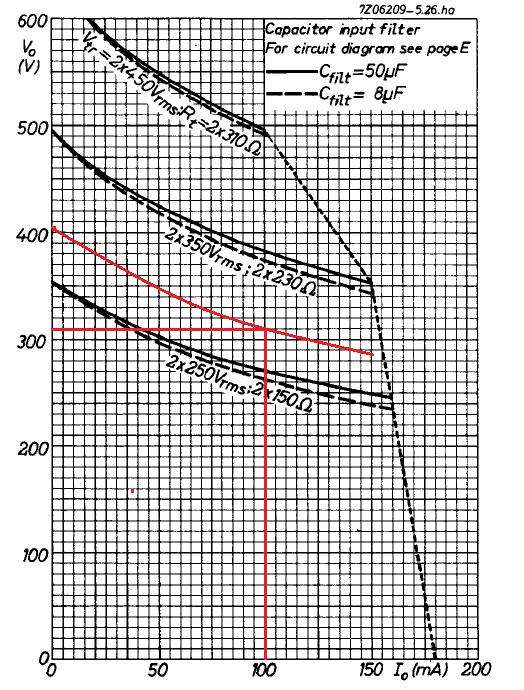# 0355st power supply

H.T. and L.T. current and voltage requirements

High tension:

Idc per channel = UV2k / R13 = 28 V / 560 Ω = 0.05 A <> 50 mA

Udc = 310 V
Idc = 2 x 50 = 100 mA

Low tension:

 Tube Uf If Qty. EF86 6.3 V 0.2 A 2 EL84 6.3 V 0.76 A 2

thus

Uf = 6.3 V
If = 2 x (0.2 + 0.76) = 1.92 A

Rectifier elements specifications

It is convenient to use a full-wave rectifier with capacitor input filter.We require 100 mA at 310 V. One of the rectifier tubes having the proper rating is the EZ81 [Datasheet] with a maximum dc output current of 150 mA, a maximum plate voltage per plate of 350 V rms, a peak inverse voltage of 1300 V, and a cathode to heater voltage of 500 V. This tube has the same shape and socket of the other amplifier tubes.

The maximum capacity of the input capacitor of smoothing filter for this tube is 50 µF. The capacitor working voltage must be at least the peak transformer output voltage.

The EZ81 heating specifications are Uf = 6.3 V and If = 1.0 A.

If we connect the heater of the EZ81 to the low-tension circuit of the other tubes (and not in a separate transformer low-tension heating winding), the low voltage requirements are

Uf = 6.3 V
If = 1.92 + 1 = 2.92 A.

And the total drawing power of the circuit is

Pt = 310 x 0.1 + 6.3 x 2.92 = 49.40 VA

We need a transformer with a minimum of 50 VA and with a high-tension secondary voltage to be determined with the help of the following EZ81 load graph.We need to supply 100 mA at 310 V. Hence, we mark the point 100, 310 in our graph, and we draw a parallel curve of the 2 x 350 V and 2 x 250 V curves that includes that point.

This curve that we have drawn intersects the abscissa axis (Io = 0) in Vo = 405 V. Which determines a no-load transformer peak voltage of 405 V, and

Us = 405 / √2 = 286 V rms

Next, we determine the minimum value for the anode protection resistor Rt at this transformer output voltage from the following graphFor a secondary transformer voltage of 2 x 286 V rms the value of Rt must be at least 180 Ω.

With the data obtained we select the power transformer from the follow list provided by Hammond Manufacturing

 Part No. VA Voltage RMS no load Voltage RMS Fil-1 Fil-2 Size 370BX 55 VA 2x 300.7 V 2x 275V @ 58mA 6.3V @ 2A 5V @ 2A X7 370CX 59 VA 2x 304.4 V 2x 275V @ 75mA 6.3V @ 2.5A 6.3V @ 0.6A X7 370DX 86 VA 2x 302.7 V 2x 275V @ 104mA 6.3V @ 3A 5V @ 2A X8 370DAX 76 VA 2x 286.8 V 2x 260V @ 104mA 6.3V @ 3.5A X8 372BX 98 VA 2x 323.1 V 2x 300V @ 115mA 6.3V @ 3A 5V @ 2A X10

Our choice is the 370DAX, which has the proper specifications

 Parameter We require 370DAX Power (VA) 50 76 Filament 6.3 V current (A) 2.92 3.5 H.T. Voltage no-load (V) 2 x 286 2 x 287 H.T. Current (mA) 100 dc 104 ac

If the load should be removed accidentally, the voltage will rise to the peak transformer high tension voltage

Um = √2 · 287 = 406 V

The working voltage of the capacitor input filter must be at least 406 V.

We can connect the EZ81 heater to the filament circuit of the other tubes, whose transformer center tap is connected to 0 V.  Since the insulation cathode to heater of the EZ81 is 500 V.

The peak inverse voltage is

Uim = 2 · Um = 2 · 406 = 812 V

Since the rated peak inverse voltage of the EZ81 tube is 1300 V, it is safe to use.

The rectifier anode protection resistor Ra minimum value is given by

Ra = Rt – (Rs + n2 · Rp)

where

Rt : the minimum protection resistance needed per anode.
Rs : the resistance of half of the secondary winding.
Rp : the resistance of the whole primary winding.
n : the ratio of the number of turns on half of the secondary winding to the number of turns on the primary winding.

From the transformer 370DAX datasheet, the winding resistances are

11,12 Ω at whole primary winding number one (120 V).
13,10 Ω at whole primary winding number two (120 V).
222,9 Ω at whole secondary winding (2 x 287 V).

and

Rs = 222,9 / 2 = 111 Ω

Rp (for 240 V) = 11,12 + 13,10 = 24 Ω
Rp (for 120 V) = 11,12 || 13,10 = 6 Ω

n (for 240 V) = Us / Up = 287 / 240 = 1,2
n (for 120 V) = Us / Up = 287 / 120 = 2,4

Thus

Ra (for 240 V) = 180 – (111 + 1.22 · 24) = 34 Ω

if still in doubt about whether the value depends on the mains voltage, a second calculation shows

Ra (for 120 V) = 180 – (111 + 2.42 · 6) = 34 Ω

If we assume the average current per resistor to be 100 / 2 = 50 mA, the dissipated power per resistor is given by

P = I2 · R = 0.052 · 34 = 0.085 W

But we have finally decided to use 33 Ω, 3 W, wire wound resistors, to give it a retro aspect.